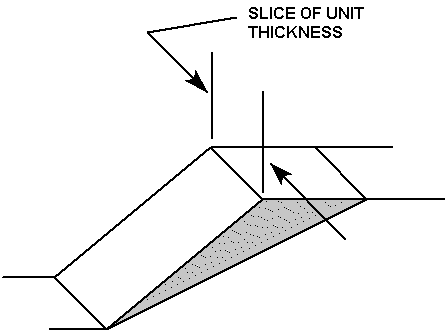The Rocscience International Conference 2021 Proceedings are now available. Read Now

# Program Assumptions

RocPlane assumes the following geometrical conditions:

• A sliding or failure plane that strikes parallel or approximately parallel (within 20 degrees) to the face of the slope.
• The failure plane daylights into the face of the slope. This condition occurs when the failure surface dips at angle shallower than the slope face.
• The presence of release surfaces at the lateral boundaries of the slide block that have insignificant resistance to sliding.The release surfaces of a sliding block

In RocPlane, the slope slice analyzed is taken perpendicular to the face of the slope, and is assumed to have unit thickness.RocPlane performs the limit equilibrium analysis of a sliding block. The Factor of Safety of the slope or sliding mass is defined as the ratio of the total forces resisting down-slope sliding to the total forces inducing sliding. The resisting forces comprise the shear strength of the sliding surface, artificial reinforcement of the slope, or other stabilizing external forces, if present. The driving forces consist of the down-slope component of the weight of the sliding block, forces generated by seismic acceleration, forces due to water pressures acting on various faces of the block, and external forces on the upper slope surface.

The limit equilibrium model in RocPlane assumes that all forces operating on a sliding block act through the centroid of the block; it ignores overturning moments. When an analysis involves a tension crack, it is assumed that the tension crack, just like the failure plane, strikes parallel to the slope face.

While many planar wedge analysis programs consider only vertical tension cracks, RocPlane allows for non-vertical tension cracks as well. Non-vertical tension cracks in RocPlane can have angles of inclination from the horizontal that are greater or less than 90 degrees.